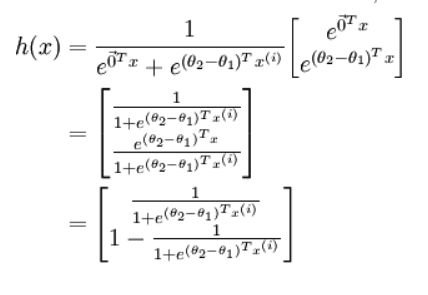# Thinkwee's Blog

Too Stupid to Give Up Learning

0%

# 统计学习方法概论

## 统计学习，监督学习，三要素

• 如果一个系统能够通过执行某个过程改进它的性能，这就是学习
• 统计学习的方法是基于数据构建统计模型从而对数据进行预测和分析
• 得到训练数据集合；确定包含所有可能模型的假设空间；确定模型选择的准则；实现求解最优模型的算法；通过学习方法选择最优模型；利用最优模型对新数据进行预测或分析
• 监督学习的任务是学习一个模型，是模型对任意给定的输入，对其相应的输出做出一个好的预测
• 每个具体的输入是一个实例，通常由特征向量表示。构成特征空间，每一维对应一个特征
• 监督学习从训练数据集合中学习模型，训练数据由输入与输出对构成(样本)
• 监督学习从训练集中学习到一个模型，表示为条件概率分布或者决策函数
• 统计学习三要素:方法=模型+策略+算法。模型包括概率模型(条件概率)和非概率模型(决策函数)；策略即选择最优模型的方法，引入损失函数(代价函数)，风险函数的概念，实现经验风险或者结构风险最优化；算法是指学习模型的具体计算方法
• 损失函数用来度量预测值相比真实值的错误程度，常见的有:0-1损失函数，平方损失函数，绝对损失函数对数损失函数，记为$$L(Y,P(Y|X))$$,风险函数(期望损失)是模型在联合分布的平均以下的损失：$R_{exp}(f)=E_p[L(Y,f(X))]=\int_{x*y}L(y,f(x))P(x,y)dxdy$经验风险(经验损失)是模型关于训练集的平均损失:$R_{emp}(f)=\frac 1N \sum_{i=1}^NL(y_i,f(x_i))$
• 理想情况下，可以用经验风险估计期望风险，然而样本容量很小时，经验风险最小化易导致过拟合，从而提出了结构风险(正则化)：$R_{srm}(f)=\frac1N \sum_{i=1}^NL(y_i,f(x_i))+ \lambda J(f)$,其中J(f)为模型的复杂性，系数$$\lambda$$用来权衡经验风险和模型复杂度。ML属于经验风险最小化，MAP属于结构风险最小化

## 模型评估，模型选择

• 模型在训练集和测试集上的误差分别称为训练误差和测试误差，所用的损失函数不一定一致，让两者一致是比较理想的
• 过拟合:学过头了，模型的复杂度比真实模型要高，只对学过的数据性能良好，对未知数据的预测能力差。避免过拟合需要正确的特征个数和正确的特征向量
• 模型选择的两种方法：正则化和交叉验证

## 正则化，交叉验证

• 即在经验风险上加一个正则化项(罚项)，模型越复杂惩罚越高
• 一般方法是将数据集随机的分成三部分:训练集、验证集、测试集，分别用来训练数据，选择模型，最终对学习方法的评估。交叉验证是将数据反复随机切分为训练集和测试集，学习多次，进行测试和模型选择
• 交叉验证类型:简单交叉验证;S折交叉验证;留一交叉验证

## 泛化能力

• 泛化误差:对未知数据预测的误差
• 泛化误差上界，一般是样本容量的函数，当样本容量增加时，泛化上界趋于0，是假设空间容量越大，模型就越难学，泛化误差上界就越大
• 对于二分类问题，泛化误差上界：其中d是函数集合容量，对任意一个函数，至少以概率$$1-\delta$$

$R_{exp}(f)\leq R_{emp}(f)+\varepsilon (d,N,\delta ) \\ \varepsilon (d,N,\delta )=\sqrt{\frac 1{2N}(log d+log \frac 1 \delta)} \\$

## 生成模型，判别模型

• 生成方法由数据学习联合概率分布，然后求出条件概率分布作为预测的模型，即生成模型，比如朴素贝叶斯法和隐马尔科夫模型
• 判别方法由数据直接学习决策函数或者条件概率分布作为预测的模型，即判别模型，比如k近邻法、感知机、决策树、逻辑斯蒂回归模型、最大熵模型、支持向量机、提升方法和条件随机场等
• 生成方法可以还原联合概率分布，学习收敛速度快，适用于存在隐变量的情况
• 判别方法准确率高，可以抽象数据，简化学习问题

## 分类，标注，回归

• 分类，即输出取离散有限值，分类决策函数也叫分类器
• 对于二分类，四种情况的总数:对的预测成对的TP;对的预测成错的FN；错的预测成对的FP；错的预测成错的TN $精确率:P=\frac{TP}{TP+FP} \\ 召回率:R=\frac{TP}{TP+FN} \\ 1F值:\frac {2}{F_1}=\frac1P+\frac1R \\$
• 标注:输入一个观测序列，输出一个标记序列
• 回归：函数拟合，常用的损失函数是平方损失函数，利用最小二乘法拟合

# k近邻法

## k近邻法

• k近邻法假设给定一个训练数据集，其中的实例类别已定。分类时，对新的实例，根据其k个最近邻的训练实例的类别，通过多数表决等方式进行预测。
• k值选择，距离度量以及分类决策规则是k近邻法三要素。
• k近邻法是一种懒惰学习，他不对样本进行训练。

## k近邻算法

• 对新的输入实例，在训练数据集中找到与该实例最邻近的k个实例，这k个实例的多数属于某个类，就把该实例分到这个类。即: $y=arg \max_{c_j} \sum_{x_i \in N_k(x)} I(y_i=c_j), \ i=1,2,...,N; \ j=1,2,...,K$ 其中$$y_i=c_i$$$$I=1$$$$N_k(x)$$是覆盖x的k个近邻点的邻域。

• k=1时称为最近邻算法

• k近邻算法没有显式的学习过程

## k近邻模型

• k近邻模型即对特征空间的划分。

• 特征空间中，对于每个训练实例点，距离该点比其他点更近的所有点组成一个区域，叫做单元。每一个训练实例点拥有一个单元，所有训练实例点的单元构成对特征空间的一个划分，每个实例的类是其单元中所有点的类标记。

• 距离度量包括：欧氏距离，$$L_p$$距离，Minkowski距离。

• 欧氏距离是$$L_p$$距离的一种特殊情况(p=2)，p=1时即曼哈顿距离，定义为： $L_p(x_i,x_j)=(\sum _{l=1}^n |x_i^{(l)}-x_j^{(l)}|^p)^{\frac1p}$

• k值较小，近似误差小，估计误差大，整体模型复杂，容易发生过拟合。k值较大，估计误差小，近似误差大，模型简单

• 一般采用交叉验证法确定k值

• 多数表决规则等价于经验风险最小化

## kd树

• kd树是用来存储训练数据的特殊结构，提高了k近邻搜索的效率，其实就是一种二叉查找树
• kd树的每一个节点对应于一个k维超矩形区域
• 构造平衡kd树的方法:依次对各个维度上取中位数进行划分，例如三维，先以x轴上中位数划线，分为两部分，每一个部分在以y轴中位数划线，然后再z轴，然后再x,y,z循环。
• 构造完成kd树后就可以利用kd树进行k近邻搜索，以下用最近邻搜索为例，即k=1：
• 从根节点出发，递归向下搜索目标点所在区域，直到叶节点
• 以此叶节点为当前最近点，当前最近点到目标点的距离是当前最近距离，我们的目标就是在树中搜索，找到合适的节点更新当前最近点和当前最近距离
• 递归向上回退，对每个节点做如下操作
• 如果该节点保存的实例点比当前最近点距离目标点更近，则更新该实例点为当前最近点，更新该实例点到目标点的距离为当前最近距离
• 该节点的子节点分为两个，其中一个包含了我们的目标点，这一部分我们是从目标点一路向上递归过来的，已经更新完了，因此要找该节点的另外一个子节点，看看可不可以更新：检查另一子节点对应区域是否与以目标点为球心，当前最近距离为半径的超球体相交，相交的话就移动到这个子节点，然后接着向上搜索，不相交则不移动，依然接着向上搜索。
• 直到搜索到根节点，此时的当前最近点即目标点的最近邻点。
• kd树搜索的计算复杂度是$$O(logN)$$，适用于训练实例数远大于空间维数的k近邻搜索

# 支持向量机

## 线性可分支持向量机与硬间隔最大化

• 学习的目标是在特征空间中找到一个分离超平面，能将实例分到不同的类(二分类)，分离超平面对应于方程$$wx+b=0$$，由法向量w和截距b决定，可由(w,b)表示
• 这里的x是特征向量$$(x_1,x_2,...)$$，而y是特征向量的标签
• 给定线性可分训练数据集，通过间隔最大化或者等价的求解相应的凸二次规划问题学习得到的分离超平面为$$wx+b=0$$，以及相应的分类决策函数$$f(x)=sign(wx+b)$$称为线性可分支持向量机
• 在超平面$$wx+b=0$$确定的情况下，点(x,y)到超平面的距离可以为 $\gamma _i=\frac{w}{||w||}x_i+\frac{b}{||w||}$
• $$wx+b$$的符号与类标记y的符号是否一致可以表示分类是否正确，$$y=\pm 1$$，这样就可以得到几何间隔 $\gamma _i=y_i(\frac{w}{||w||}x_i+\frac{b}{||w||}) \\ 定义超平面(w,b)关于训练数据集T的几何间隔为关于所有样本点的几何间隔的最小值 \\ \gamma=min\gamma _i \\$
• 同时定义相对距离为函数间隔 $\gamma _i=y_i(wx_i+b) \\ \gamma =min\gamma _i \\$
• 硬间隔最大化针对线性可分超平面而言，软间隔最大化针对近似线性可分而言
• 求一个几何间隔最大的分离超平面，可以表示为下面的约束最优化问题 $max_{(w,b)} \gamma \\ s.t. \quad y_i(\frac{w}{||w||}x_i+\frac{b}{||w||})\geq \gamma ,i=1,2,...,N \\$
• 我们可以将几何间隔转化为函数间隔，对最优化没有影响，同时，如果我们固定相对间隔为常量(1)，则对几何间隔的最大化可以转化为对$$||w||$$的最小化，因此约束最优化问题可以改写为 $min_{(w,b)} \frac12 {||w||}^2 \\ s.t. \quad y_i(wx_i+b)-1 \geq 0 \\$
• 上式就是SVM的基本型,当解出以上最优化问题时，我们就得到了一个拥有最大间隔的分离超平面，这就是最大间隔法
• 最大间隔分离超平面存在且唯一，证明略
• 在线性可分情况下，训练数据集的样本点中分离超平面最近的样本点的实例称为支持向量
• $$y_i=1$$的正例点，支持向量在平面$$wx+b=1$$上，同理负例点在平面$$wx+b=-1$$上，这两个平面平行，之间没有任何训练数据点，两个平面的间隔距离为间隔(margin)，间隔依赖于分离超平面的法向量w，为$$\frac 2 {||w||}$$
• 由此可见支持向量机由很少的重要的训练样本(支持向量)决定
• 为了解最优化问题，我们引入对偶算法，同时引入核函数以便推广到非线性分类问题
• 待补充

# 线代基础

## Moore-penrose

• 对于非方矩阵，其逆矩阵没有定义，因此我们特别定义非方矩阵的伪逆：Moore-Penrose 伪逆 $A^+=lim_{\alpha \rightarrow 0}(A^TA+\alpha I)^{-1}A^T$
• 计算伪逆的实际算法没有基于这个定义，而是使用下面的公式： $A^+=VD^+U^T$ 其中U、D、V是矩阵A奇异值分解后得到的矩阵，对角矩阵。对角矩阵 D 的伪逆$$D^+$$是其非零元素取倒数之后再转置得到的。
• 当矩阵 A 的列数多于行数时，使用伪逆求解线性方程是众多可能解法中的一种。特别地，$$x = A^+y$$是方程所有可行解中欧几里得范数最小的一个。
• 当矩阵 A 的行数多于列数时，可能没有解。在这种情况下，通过伪逆得到的$$x$$使得$$Ax$$$$y$$的欧几里得距离最小。
• 待补充

## 迹

• 迹运算返回的是矩阵对角元素的和.
• 使用迹运算可以描述矩阵Frobenius范数的方式： $||A_F||=\sqrt{Tr(AA^T)}$
• 迹具有转置不变性和轮换不变性
• 标量的迹是其本身

• 待补充

# 概率论信息论

## Logistic Sigmoid

• Logistic和sigmoid两种称呼经常混用，这个函数用于将实数压缩到(0,1)之间，代表二分类概率： $\sigma (x) = \frac{1}{1+exp(-x)}$
• Softmax 是sigmoid的扩展版，是argmax函数的软化版本（argmax返回一个one hot 向量而softmax返回的是各种可能的概率），将二分类扩展到多分类（互斥）情况： $\sigma (z)_j = \frac{e^z j}{\sum _{k=1}^K e^z k}$
• 两者在输入过大过小时都存在饱和现象，但将两个函数作为非线性激活单元引入神经网络时，因为代价函数是取负对数，可以消除这种饱和现象。
• Softmax函数因为包含指数函数，还存在上下溢出问题。当输入均匀分布且输入样本数量很大时，分母指数值接近于0，累加也可能接近于0，导致分母下溢。当指数函数参数很大时也会导致上溢。解决办法是将输入x处理为z=x-max(xi)，即向量的每个分量都减去最大分量，输入向量加减标量不会导致softmax函数值改变(softmax函数的冗余性），但此时输入经处理后最大值为0，排除上溢，经过指数函数后分母的累加项中至少存在一个1，排除下溢。
• 利用softmax函数的冗余性也可以推出sigmoid是softmax的一种特例：## KL散度和交叉熵

• KL散度：用以衡量PQ两个分布之间的差异，非负且不对称： $D_{KL}(P||Q) = E_{x \sim P} [log \frac{P(x)}{Q(x)}] = E_{x \sim P} [log P(x) - log Q(x)]$
• 交叉熵： $H(P,Q) = -E_{x \sim P} log Q(x)$
• 交叉熵形式简单，而且针对Q（实际输出）最小化KL散度与散度公式中前一项无关系，因此最小化KL散度实际上可以看成最小化交叉熵，又因为KL散度代表PQ（实际输出和正确输出）之间的差异，即可以看作是损失函数
• 在用logistic处理二分类问题中，q(x)即logistic函数,p(x)即实际数据的正确分布(0或者1)
• 对q按照p求自信息期望即二元交叉熵(Logistic代价函数): $J(\theta) = - \frac 1m [\sum _{i=1}^m y^{(i)} log h_{\theta} (x^{(i)}) + (1-y^{(i)}) log (1-h_{\theta}(x^{(i)}))]$
• 同理可得多元交叉熵(Softmaxs代价函数): $J(\theta) = - \frac 1m [\sum _{i=1}^m \sum _{j=1}^k 1\{ y^{(i)}=j \} log \frac {e^{\theta _j ^T x^{(i)}}} {\sum _{l=1}^k e^{\theta _j ^T x^{(i)}}}]$

## 交叉熵与最大对数似然关系

• 已知一个样本数据集X，分布为$$P_{data}(x)$$，我们希望得到一个模型$$P_{model}(x,\theta)$$，其分布尽可能接近$$P_{data}(x)$$$$P_{model}(x,\theta)$$将任意x映射为实数来估计真实概率$$P_{data}(x)$$。 在$$P_{model}(x,\theta)$$中，对$$\theta$$的最大似然估计为使样本数据通过模型得到概率之积最大的$$\theta$$$\theta _{ML} = \mathop{argmax}\limits_{\theta} p_{model} (X;\theta)$
• 因为取对数和尺度变换不会改变argmax，取对数变累加并除以样本数量平均后得到： $\theta _{ML} = \mathop{argmax}\limits_{\theta} E_{x \sim p_{data}} log p_{model}(x;\theta)$
• 可以发现上式即交叉熵的相反数，当Pdata(x)=Pmodel(x,θ)时上式值最大，所以:
• 最大似然=最小负对数似然=最小化交叉熵=最小化KL散度=最小化数据与模型之间的差距∈最小化代价函数
• 最大似然估计可扩展到最大条件似然估计，构成了大多数监督学习基础：公式： $\theta _{ML} = \mathop{argmax}\limits_{\theta} \sum_{i=1}^m log P(y^{(i)} | x^{(i)} ; \theta)$
• 最大似然估计具有一致性。

# 计算方法

## 梯度下降

• 问题：为了使函数变小（最小化代价函数），怎样变换参数（输入）？
• 原理：将输入向导数的反方向移动一小步可以减小函数输出。
• 将输入扩展到向量形式的参数，将函数看成代价函数，即得到基于梯度的优化算法。
• 一阶优化算法：包括梯度下降，使用Jacobian矩阵（包含向量之间偏导数关系），通过梯度下降对参数的建议更新为：

## 牛顿法

• 二阶优化算法：（求最优补偿，定性临界点）:一阶优化需要调整合适的学习率（步长），否则无法达到最优点或者会产生抖动，且在临界点（梯度为0）无法更新参数，这反映我们需要代价函数的二阶导数信息，例如函数向上凸出或向下凸出时基于梯度的预测值和真实的代价函数值之间有偏差。Hessian矩阵包含了二阶信息。牛顿法使用了Hessian矩阵的信息，利用泰勒二阶展开得到函数信息，利用下式更新参数： ## 约束优化
• 只包含等式约束条件：Lagrange
• 包含不等式约束条件：KTT

# 修改算法

## 修改假设空间

• 机器学习算法应避免过拟合和欠拟合，可以通过调整模型容量（拟合各种函数的能力）来解决。
• 调整模型容量的方案是选择合适的假设空间（假设输入而不是参数），例如之前只拟合多项式线性函数： $y = b + wx$
• 如果引入非线性单元，例如高次项，输出相对于参数仍然是线性分布： $y= b + w_1 x + w_2 x^2$ 此时就增加了模型的容量，但简化了生成的参数，适用于解决复杂的问题，然而容量太高也有可能过拟合。

## 正则化

• 没有免费午餐定理（在所有可能的数据生成分布上平均之后，每一个分类算法在未事先观测的点上都有相同的错误率）说明应在特定任务上设计机器学习算法，算法应该有偏好。将代价函数中加入正则化即引入偏好，使得学习到的参数偏向于使正则化项变小。
• 一个例子是权重衰减，加入权重衰减正则化项的代价函数是： $J(w) = MSE_{train} + \lambda w^T w$ λ控制偏好程度，生成的模型倾向于小参数，可以避免过拟合。